# Money Management-Section 7: Fixed Ratio

## Section 7: Fixed Ratio

This technique was developed by Ryan Jones. He has written a whole book but we shall try to condense the main principles into a few paragraphs. It states that the relationship between the number of contracts being traded and the amount of profits required to increase to an additional contract should remain fixed. It gets a bit technical so we have tried to simplify as best we could with examples.

This means that if the MM required \$5,000 in profits trading only one contract to increase to two contracts, then it should require \$10,000 in profits trading two contracts to increase from two to three contracts. With us so far?

This methodology uses one variable, what Ryan Jones calls delta. This variable determines how aggressively or conservatively the MM is applied. The lower the delta, the more aggressive the MM is, the higher the delta, the more conservative the MM is. If you are interested DELTA is the fourth letter of the Greek alphabet and means a small variable

The formula to calculate the levels in which the trader should increase contracts is calculated as follows:

Previous required equity + [# of contracts traded * delta] = Next level in which the trader should increase contracts.

For instance, in a \$50,000 trading account and a delta equal to \$5,000

\$50,000 + (1 * 5,000) = \$55,000. This means that at \$55,000 we should increase our trading size from one contract to two contracts

The next calculation starts at \$55,000

\$55,000 + [2 * \$5,000] = \$65,000. When we reach \$65,000 we should increase our trading size to three contracts.

And so on.

What would happen to our profits in a streak of winning trades with different deltas?

Take profit level fixed at \$3,000 in a \$50,000 trading account.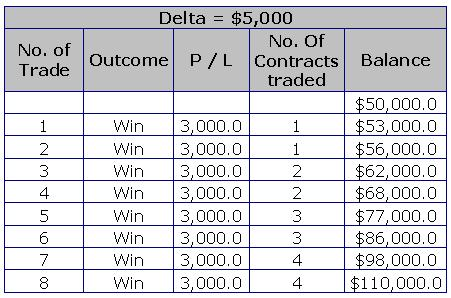[Table 1]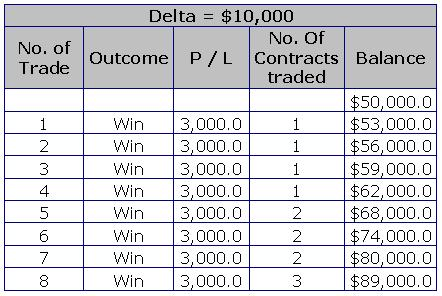[Table 2]

But, what would happen if a drawdown occurs before any winning trade? It would have the same results than using no money management at all. Ryan Jones does not mention what to do in this case.

The best thing to do is to use another MM technique should this happen. For instance, we could use a fixed fractional approach, but as soon as we recover back the losses, apply again the fixed ratio approach.

Now let us take a look at the results using the same sequence of trades used with the other MM techniques.[Table 3]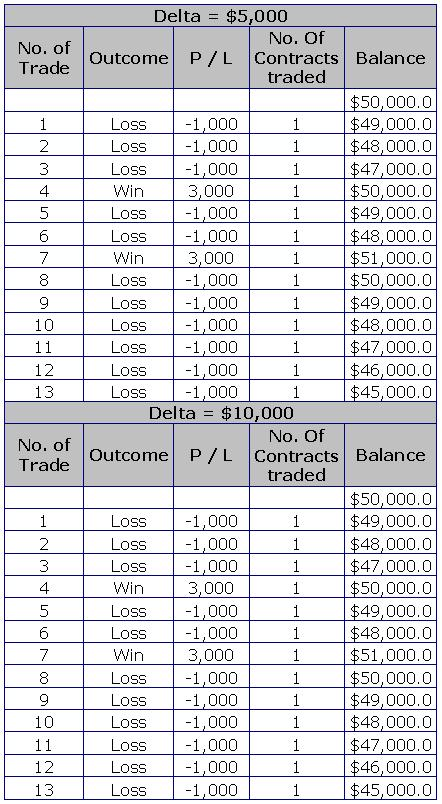[Table 4]

As you can see, the results with both deltas is the same, the reason for this is because the fixed ratio technique never makes it to trade two contracts with either delta.

Now, let us take a look at the consecutive winning streak.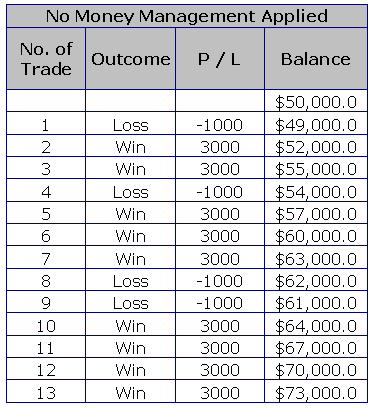[Table 5]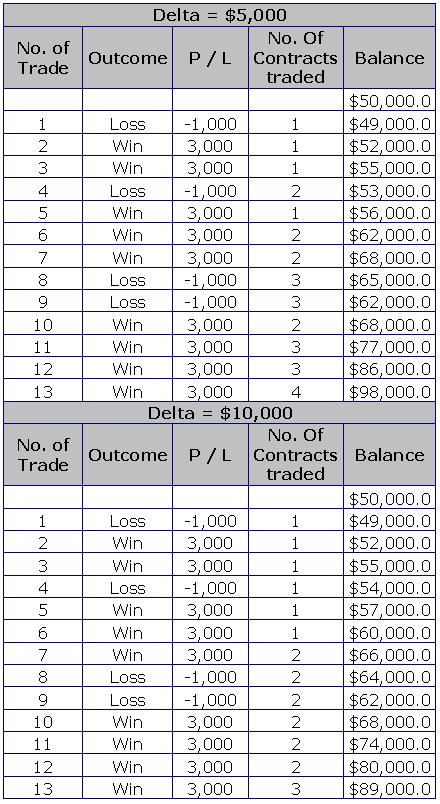[Table 6]

As we can see, the difference is self-evident. Using a delta of \$5,000 the balance ends up at \$98,000 and with a delta of \$10,000, the balance ends up at \$89,000 against \$73,000 when no MM is applied.

We have seen several MM techniques by now, but which one is better?

It depends on each trader, some techniques are excellent on the risk side and some others are excellent on the profits side. Therefore, it is up to the trader to decide which MM techniques to use and the parameters to be used.

What we are certain about is that using a MM technique will put the odds in your favor. On the other side, not using a MM technique would put every trader through a hard time with only a slim chance to succeed in this business. So I hope we have managed to convince you to adopt MM. Some of the traders we have taught try a few out to see which one they like before deciding on one and sticking to it – up to you.